current position: Home > News

#### Design of Wilkinson RF Power Divider

2022-02-16 10:33:23 in Technology | Power Divider Tech

Design of S-Band Combline Coaxial Cavity Bandpass Filter

This paper presents the design of high power, low loss combline coaxial cavity bandpass filter. The design is based on Chebyshev prototype element values for lowpass filters, which are used in calculating the normalized capacitances per unit length between adjacent resonators and resonator to ground. The resonator diameter depends on the capacitance between  resonator and the ground, while its length is set equal to λo/8, where λo is the free space wavelength at center frequency fo. Similarly the spacing between resonators is calculated using the capacitances between adjacent resonators. In order to tune the filter fo and bandwidth, the tuning and coupling screws are added in the design. The diameter of screws is computed using coaxial capacitance formulations. Once the theoretical calculations are completed, the filter is optimized using 3-D EM simulator to finalize its dimensions. A 5th-order prototype filter at 2.8 GHz with 9% FBW (fractional bandwidth) and 0.01 dB passband ripple is designed, optimized and fabricated for verification. A good agreement between the measured and simulated results validates the proposed synthesis technique.

Index Terms— Bandpass filter, combline, coaxial resonator, cavity filter, S-band.

I.                    INTRODUCTION

BANDPASS filters with low insertion loss and high selectivity are widely used in modern communication systems, to isolate the desired frequencies from unwanted signals. Microwave cavity filters are preferred over distributed counterparts, where narrowband, high selectivity and high power handling are required, especially in radar and satellite transceivers. In recent years a lot of research has been done to develop low loss coaxial cavity filters. The coaxial cavity filters using different spatial orientation of resonators to create cross couplings are discussed in “True inline cross-coupled coaxial cavity filters" and “Orthogonal  coaxial  cavity  filters  with distributed cross-coupling". Furthermore, the size  of filter is reduced by adding the capacitance circular plate on resonant rod “Design of a C-band coaxial cavity band  pass filter", forming a stepped impedance structure. Another synthesis technique which employs hybrid dielectric and coaxial resonators “Spurious-free differential bandpass filter using hybrid dielectric and coaxial resonators" , results in wide differential-mode (DM) stopband and improved common-mode (CM) suppression. In addition a mechanically tunable combline cavity filter replacing the rod-tuning screw combination with printed circuit-tuning screw combination is introduced in “Novel microstrip  fed  mechanically  tunable combline cavity filter", which results in small size, less weight, integration with other printed circuits and significant reduction in fabrication complexity. A reconfigurable combline cavity filter formed by cascading two asymmetrical filters is designed in “A reconfigurable microwave combline filter" .

This paper covers the design, development and testing of S- band inline combline coaxial cavity filter. The metallic cavity and high Q (quality factor) coaxial resonators gives the advantages of narrowband, low loss, high selectivity and high power handling capability when compared to microstrip  filters. Furthermore, the tuning and coupling screws allows for post manufacturing frequency and bandwidth adjustment. The paper is arranged as follows. Section II gives the detail mathematical   design of the th-order filter.Section III addresses the 3-D EM simulations, hardware realization and measured vs simulated result's comparison. Section IV provides the conclusion.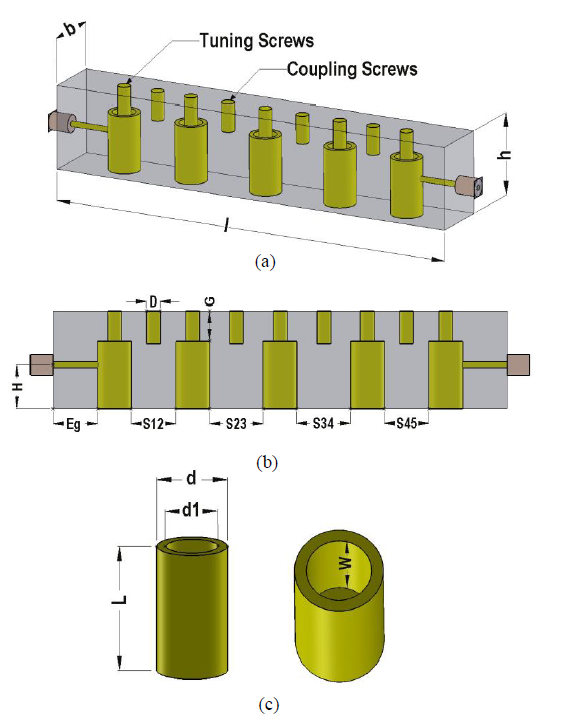Fig.1. Filter cavity and resonator models. (a) 3-D model with cavity dimensions. (b) 2-D modal with filter parameters. (c) Coaxial resonator

II.        FILTER DESIGN

The proposed S-band filter has fo=2.8 GHz, FBW=9%, passband ripple=0.01dB and 3 dB rejection at fo +300 MHz. The  Chebyshev attenuation characteristics plot for 0.01dB ripple  "Microwave filters, impedance-matching networks, and coupling structures", gives the order n=5  for the desired filter.Once the order is finalized.Fig.1 shows the 3-D simulation model of the filter along with 2-D view and coaxial resonator sturcture. The resonator length 'L’is set equal to λo/8. The resonator diameter 'd’is calculated from the normalized capacitance per unit length between resonators and ground, while normalized capacitance per unit length between adjacent resonators, gives the distances 'Sij’, where indexes 'i’ and 'j’indicate the resonator  number. The combline normalized capacitances are computed using the Chebyshev prototype g-values for lowpass filter. Table 1 gives the g-values for 5th-order filter “Microwave filters, impedance-matching networks, and coupling structures”, with 0.01 dB passband ripple.

TABLE 1

Element Values for Chebychev Filter with n=5 and 0.01dB Ripple

 g0 g1 g2 g3 g4 g5 g6 1.0 0.7563 1.3049 1.5773 1.3049 0.7563 1.0

A.        Normalized Capacitance between Resonators and Ground

This section gives the capacitances required for resonator 'd’ calculation. At first the resonator is assumed in rectangular form and then converted to cylindrical structure with diameter 'd’. The rectangular resonator has length 'L’, width 'w’and thickness 't’. In order to achieve high unloaded 'Q’ for filter, the resonator impedance is taken as 70 Ω. The mathematical formulations for the normalized capacitances per unit length, between resonators and ground for combline filter  "Microwave filters, impedance-matching networks, and coupling structures", are given in (1)-(5).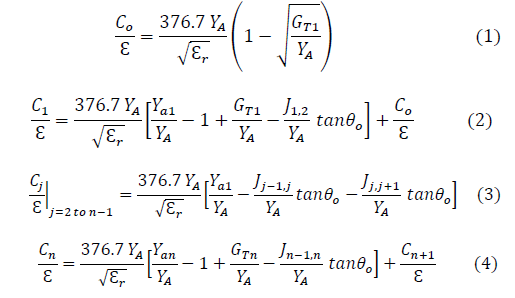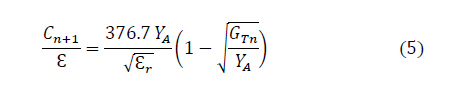where,εis the permittivity,εr=1, ‘n’ is the order of filter,Yaj is the resonator admittance (1/70) andYA= 1/50. The factorsGTYA andJj,j+1YA are calculated in (6)-(8).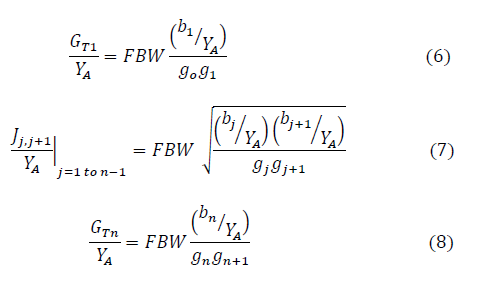where,bjYA=0.918 for λ/8 resonators "A practical design  of  lumped,  semi-lumped  and  microwave cavity filters", with impedance equal to 70 Ω.

B.        Normalized Capacitance between Adjacent Resonators

This section gives the capacitances required for the distances 'Sij' calculation. The mathematical formulas for  the normalized capacitances per unit length between adjacent resonators "Microwave filters, impedance-matching networks, and coupling structures", for combline filter are given in (9)-(11).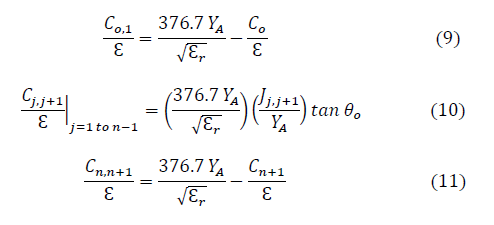where, θo=45° for λ/8 resonators. All the mathematical calculations for normalized capacitances are done by  "Microwave filters, impedance-matching networks, and coupling structures".

C.        Physical Dimensions of Filter

Once the normalized capacitance tables are created by computations,the next step is the calculation of filter dimensions indicated in Fig.1.The cavity width chosen for design in this work is b=16mm.The distance between resonators ‘Sij’ between resonators are obtained by the plots between S/b and interbar capacitanceΔC∕ε "Microwave filters, impedance-matching networks, and coupling structures",for t/b=0.2,whereΔCε=Cj,j+1∕ε,derived in (9)-(11).Eq.(12) gives the normalized width ‘Wj’ of rectangular resonators.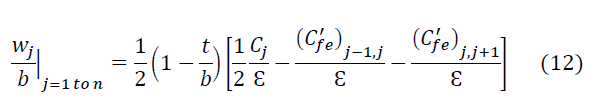where,cfⅇ'∕ε is the normalized even-mode fringing capacitance obtained by the plot betweencfⅇ'∕εand S/b,for t/b=0.2.In order to avoid the complexity of referring to the plots,this paper uses Lagrange interpolation technique with second degree polynomials to calcuate Sij/b and wj/b.The small error induced by interpolation is removed by optimization using 3-D EM simulator.The rectangular resonators are converted to cylindrical ones by equating the circumference of both type of resonators as shown in (13).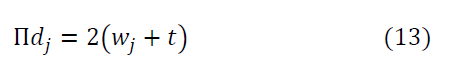where,t=3.2 mm for t/b=0.2 and b=16 mm.For simplicity and symmetry of physical structure,the diameter of each resonator is set equal to the average diameter value given by (14).Another important parameter is the end-gap (Eg),defined as the air gap between cavity wallthe end resnators,as shown in Fig.1(b).The gap selected for design in this work is Eg=5 mm.The length of cavity ‘I’ shown in Fig.1(a),can now be calcuated using (15).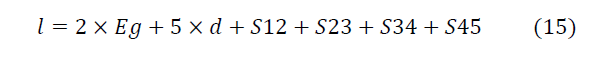After the cavity dimensions are computed,the final step is to calcuate the screw diameter ‘D’,air-gap ‘G’,depth ‘W’ and diameter ‘d1’ of the hole drilled in resonators,as shown in Figs.1(b) and 1(c).The resonators are tuned to resonate at fo,using the distributed capacitance ‘Cr’ developed at the free end of resonators.The capacitance Cr is calcuate using (16).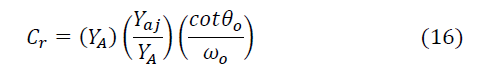The values ofYA,Yaj andθ0are given in sectionII-A,whilew0=2Πf0.Using (19),the value of capacitance,Cr=0.8 pF is obtained.The gap ‘G’ between resonator top and cavity top plays an important role in determining ‘Cr’ if parallel plate capacitance is assumed between resonator top and cavity top.However such an assumption can lead to small impractical values of ‘G’ from manufacturing point of view,therefore Cr is created using coaxial capacitance formulation.A hole of diameter ‘d1’ is drilled into resonator forming a coaxial structure with a tuning screw of diameter ‘D’ inserted into this hole.The values of ‘d1’ and ‘W’ are computed using the coaxial capacitance formula given in (17).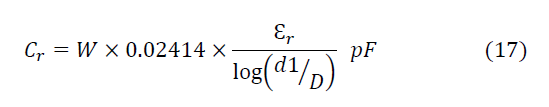Taking d1=5.5 mm,D=3 mm and W=9 mm the value of Cr=0.8 pF is obtained from (20),which is same as the value calculated from (19).Therefore the depth W=10 mm is finalized for fabrication purposes,with extra 1mm for tunning optimization.The value of air-gap is chosen as G=4 mm.There are four coupling screws in the filter as depicted in Fig.1(a),which are used for tuning the bandwidth.For symmetry purposes,the diameter of coupling screws is set equal to tuning screw diameter ‘D’.Finally the height ‘h’ of cavity is calcuated as,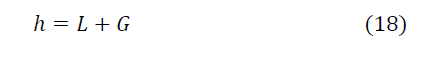TABLE 2

FILTER PHYSICAL PARAMETERS(UNITS:MM)

 Parameters Theoretical HFSS Optimized b 16 16 S12 6.6 6.5 S23 8.4 8.7 S34 8.4 8.7 S45 6.6 6.5 Eg 5 D 3 3 G 4 H 8.7 d 6.7 7.5 d1 5.5 5.5 L 13.4 13.4 W 10 10

III.     FILTER SIMULATIONS, MANUFACTURING AND EXPERIMENTAL RESULTS

After completing the theoretical design of filter,the filter is optimized using 3-D EM simulations in HFSS.The 3-D structure of the filter (Fig.1(a)),is created in HFSS and simulated using Modal solver with adaptive tetrahedral mesh.

There are two methods to connect the input/output(I/O) ports to the end resonators.One way is the use of parallel coupling probe,while the other method involves the direct connection of connector pin to the end resonators.In this work the direct soldering of connector pin to the resonators is applied as depicted in Fig.1(a).The height ‘H’ of the pin from ground to the contact point at resonator,plays a vital role in the VSWR calculation of filter response.Table 2 gives the theoretical and HFSS physical parameters of the filter.

Fig. 3 gives the comparison of simulated and measured results of the filter. All the measurements are taken using microwave network analyzer (PNA). The filter center frequency and insertion loss are tuned using tuning screws, while the bandwidth is adjusted using coupling screws. After tuning, an insertion loss of 0. 6 dB is noted at fo, while return loss better than 18 dB is obtained with in the passband. Fig. 3 also gives the enlarged view of the filter passband for close comparison. The bandwidth of the filter is set little higher than the simulated value with the help of coupling screws, to achieve low insertion loss at passband edges. This consequently reduces the stopband rejection slightly, when compared to the simulated response. Overall a good match between measured and simulated results is obtained which verifies the proposed design methodology.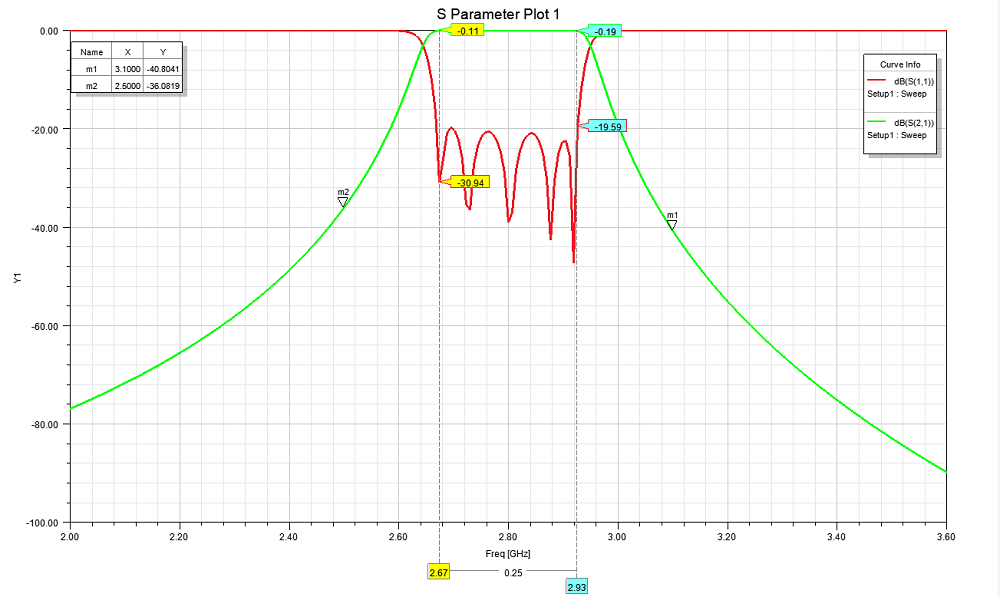Fig.3. Simulated result

IV.   CONCLUSION

The design, development and testing of high selectivity, low loss, narrowband cavity filter at S-band is presented. The proposed combline architecture has better insertion loss due to high Q coaxial cavity structure and high power handling capability due to mechanical cavity, when compared to microstrip filters. In addition, the use of tuning and coupling screws allows for the post manufacturing frequency and bandwidth tuning. The S-parameter measurements of the  tuned filter verify the intended performance.